## Common commands to deal with date in Stata

`egen compdatadate=eom(fiscalmonth fiscalyear)`
`format compdatadate %td`

To be continued …

## Stata command to order tabulation result with only top values shown

`tabulate varname` command is handy in Stata, but sometimes it returns a too long result, if `varname` contains too many unique values.

The third-party command, `groups`, will solve the problem by showing top values only. Please use `ssc install groups` to install `groups`. The usage of `group` is very similar to `tabulate`. Here are some examples:

## Empower “and” and “or” in IF statement in Stata

Stata is a little bit awkward when using `and` and `or` in `if` statement, compared to SAS. For example:

In SAS, we can write `if 2001 <= fyear <= 2010`. But in Stata, we usually write: `if fyear >= 2001 & fyear <= 2010`.

In fact, Stata provides a handy `inrange` function. The above `if` statement can be written as: `if inrange(fyear, 2001, 2010)`.

Similarly, Stata provides another `inlist` function. The syntax is `inlist(z, a, b, ...)`, which returns 1 if z = a or z = b … In `if` statement, it is equivalent to `if z = a | z = b | ...`

Posted in Stata | 1 Comment

## Display mean and median test results in Stata

Sometimes we may want to produce the following table to compare the mean and median of two groups: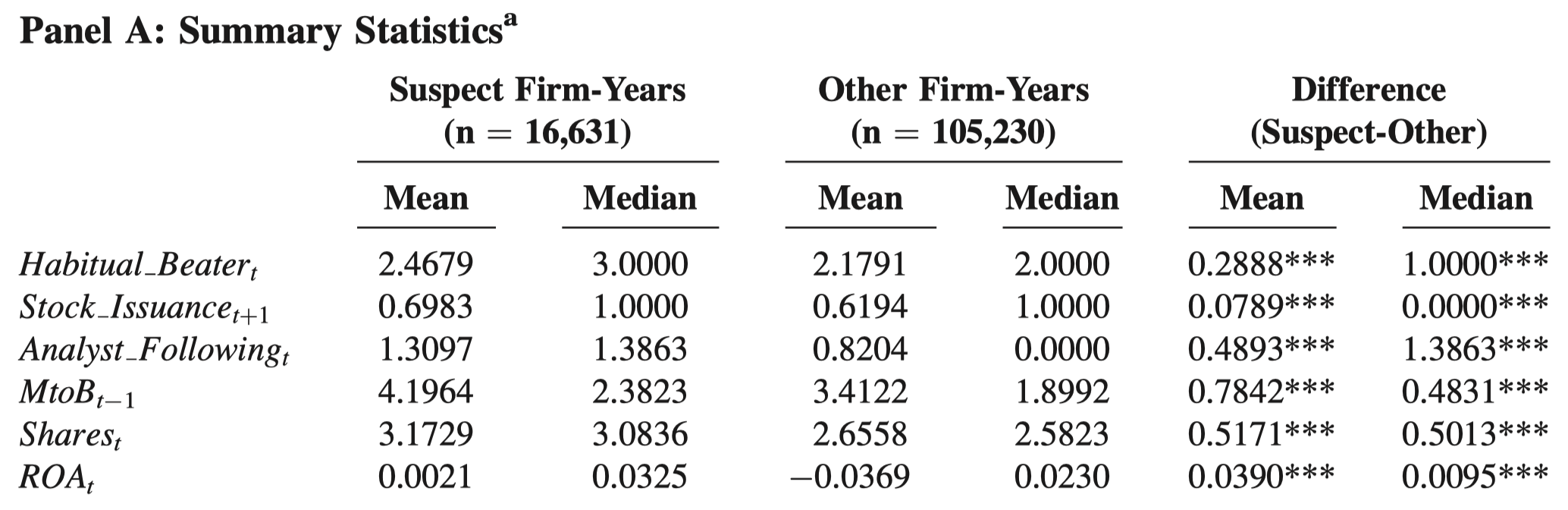First of all, please refer to this post to see Stata commands to test equality of mean and median.

However, it is time-consuming to glean numbers from the output of these Stata commands and place them in a table. It is even more struggling that you have to repeat the tedious process every time you update your sample.

I write Stata codes to streamline the process. The codes vary between unpaired (i.e., unmatched) data and paired data.

Unpaired data

The above example is unpaired data, i.e., suspect firm-years and other firm-years are not 1-to-1 or 1-to-m matched. One usage of unpaired data is the first step of Heckman’s two-step procedure, in which two groups of observations (i.e., the group that will be selected into the second step and the group that will not be selected into the second step) are stacked vertically in the dataset. The following codes are used for unpaired data. You only need to modify the first two lines to suit your data. The codes will generate a table in Stata’s output window like this: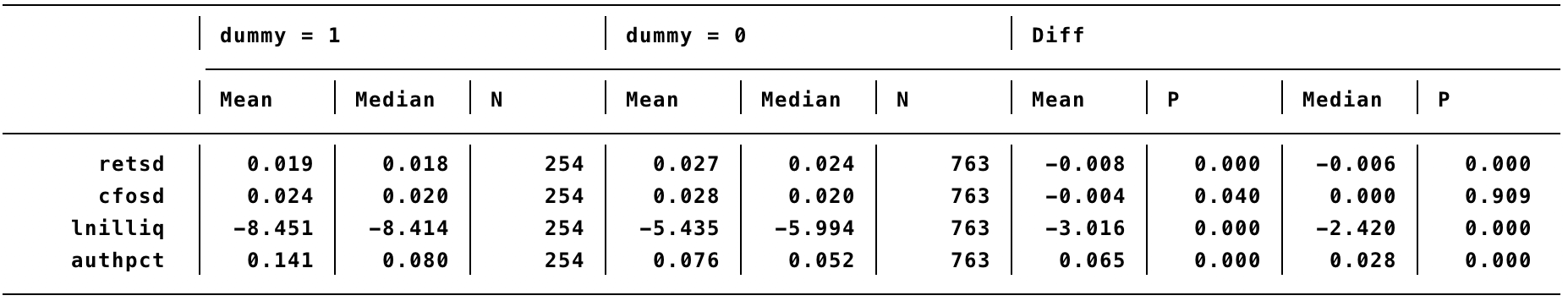You can then select the output and right-click “Copy as table” and paste in Excel for a quick edit. The codes use t-test for mean and Wilcoxon rank-sum test for median.

Paired data

A typical usage of paired data is to identify a matched control group for the treatment group. For example, identify a matched firm-year for an event firm-year based on a set of characteristics (same industry, same year, similar size and book-to-market), or identify a matched firm for every event firm based on the closest propensity score (i.e., propensity score matching).

The following table is an example that compares the mean and median of two matched groups—restating firms and non-restating group. Each restating firm is matched with a non-restating firm.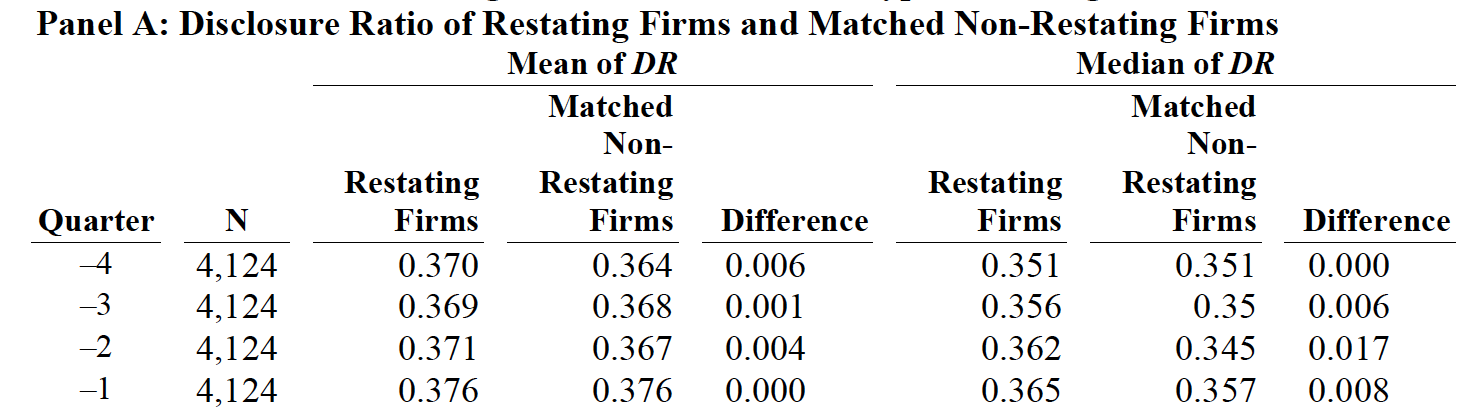Because of this matching relationship, every event firm and its control firm will be placed in the same row in the dataset. In other words, event firms and control firms are aligned horizontally. The following codes are used for paired data. You only need to modify the first two lines to suit your data. You must specify the same number of variables in the matched order in the first two lines. In other words, the first variable in the first line must be paired with the first variable in the second line, and so on. The codes will generate a table in Stata’s output window like this: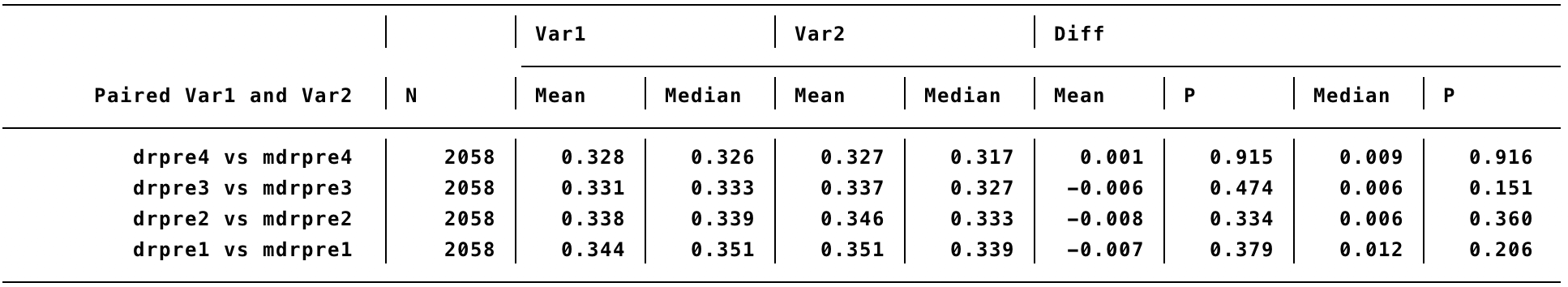The codes use paired t-test for mean and Wilcoxon rank-sign test for median.

Posted in Stata | 21 Comments

## Stata commands to do Heckman two steps

We often see Heckman’s two steps in accounting literature. But how to do it in Stata?

The two steps refer to the following two regressions:

Outcome equation: `y = X × b1 + u1`
Selection equation: `Dummy = Z × b2 + u2`

The selection equation must contain at least one variable that is not in the outcome equation.

The selection equation must be estimated using Probit. An intuitive way to do Heckman’s two steps is to estimate the selection equation first. Then include inverse mills ratio (IMR) derived from the selection equation in the outcome equation. In other words, run two regressions, one after the other.

Stata command for the selection equation:

`probit Dummy X` (using both observations that are selected into the sample and observations that are not selected into the sample, i.e., `Dummy` = 1 or `Dummy` = 0)

Note `vce` option (i.e., standard, robust or clustered standard errors, among others) will not change the resultant IMR.

Next, calculate IMR immediately:

`predict probitxb, xb`
`ge pdf = normalden(probitxb)`
`ge cdf = normal(probitxb)`
`ge imr = pdf/cdf`

Finally, include `imr` in the outcome equation:

`reg y X imr, vce(specified_vcetype)` (using observations that are selected into the sample only)

Note the first and the second regression use different numbers of observations.

However, this is not over. I find the first Probit regression sometimes causes missing IMR. For example, even if I have 100 observations with required `Dummy` and `X` data, I may only get IMR for 60 observations using this step-by-step method. I have not figured out why.

I then note that Stata in fact provides an all-in-one method to estimate both the selection equation and the outcome equation in one command `heckman`:

`heckman y X, select(Dummy = Z), twostep first mills(imr) vce(specfied_vcetype)`

I recommend using `twostep` option of the `heckman` command. This option will produce the same results with the step-by-step method. But this option may reduce the number of available `vce` types. In addition, the specified `vce` option only applies to the outcome equation and has no effect on the selection equation.

In this all-in-one method, we must pool together both observations that are selected into the sample and observations that are not selected into the sample, in which `Dummy` is 1 or 0 for all observations and `y` and `X` are missing for observations that are not selected into the sample. A benefit of this all-in-one method is that the weird missing-IMR issue will not appear.

I do have a closer look at missing IMR from the step-by-step method. They all have an extremely small value in the all-in-one method. I find that the step-by-step method has greater flexibility. Thus, if we want to use the step-by-step method but encounter the weird missing-IMR issue, it seems safe to just set missing IMR as zero.

Any comment is welcome.

Posted in Stata | 7 Comments

## The calculation of average credit rating using ratings from three rating agencies

I was doing something in Finance and wanted to calculate the average rounded credit rating. Basically, I need to translate textual grades (e.g., AAA, Baa) to a numerical value. I found a clue in the following paper:

Becker, B., and T. Milbourn. 2011. How did increased competition affect credit ratings? Journal of Financial Economics 101 (3):493-514.

See their Table 2 for an overview of the ratings levels for the three main rating agencies and the numerical value assignments used in their empirical work.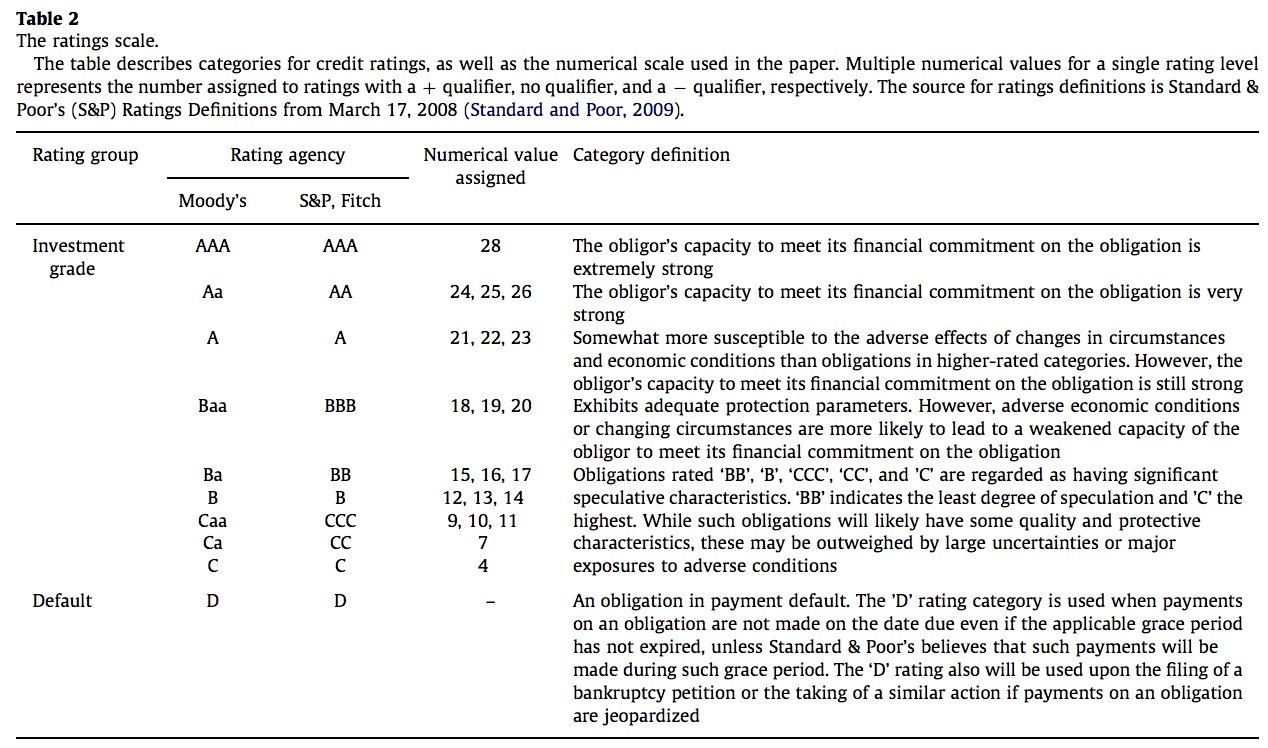Posted in Data | 1 Comment

## Stata commands to test equality of mean and median

DataCommand to Test Equality of MeanCommand to Test Equality of Median
Paired or matchedPaired t test:
`ttest var1 = var2`
Wilcoxon matched-pairs signed-rank test:
`signrank var1 = var2`

Sign test of matched pairs:
`signtest var1 = var2`
Unpaired or unmatchedTwo-sample t test:
`ttest var, by(groupvar)`
Wilcoxon rank-sum test or Mann_Whitney test:
`ranksum var, by(groupvar)`

K-sample equality-of-medians test:
`median var, by(groupvar)`

UCLA IDRE has posted an article (link) that may provide a bit more explanation. UCLA IDRE is a great resource for learning statistical analysis. A big thank you to them.

Posted in Stata | 3 Comments

## Stata command to display combined Pearson and Spearman correlation matrix

Oftentimes we would like to display Pearson correlations below the diagonal and Spearman correlations above the diagonal. Two built-in commands, `pwcorr` and `spearman`, can do the job. However, we have to manually combine Stata output tables when producing the correlation table in the manuscript, which is time-consuming.

I find this fantastic module written by Daniel Klein. His command will return one table that combines Pearson and Spearman correlations and needs the fewest further edits. Thanks Daniel and please find his work here.

A sample command is as follows:

`corsp varlist, pw sig`

To install Daniel’s module, type `ssc install corsp` in Stata’s command window.

A good technical comparison of Pearson and Spearman correlations can be found here.

Posted in Stata | 1 Comment

## Stata command to convert string GVKEY to numerical GVKEY or vice versa

The default type of GVEKY in Compustat is string. Sometimes, we need it to be a numerical type in Stata (e.g., when we want to use the super handy command `tsset`). The command to convert string GVKEY to numerical GVEKY is very simple:

`destring gvkey, replace`

The command to revert numerical GVKEY to string GVKEY with leading zeros is as follows:

`tostring gvkey, replace format(%06.0f)`

Posted in Stata | 1 Comment

## Stata command to calculate the area under ROC curve

If we want to evaluate the predictive ability of a logit or probit model, Kim and Skinner (2012, JAE, Measuring securities litigation risk) suggest that

A better way of comparing the predictive ability of different models is to use the Receiver Operating Characteristic, or ROC curve (e.g., Hosmer and Lemeshow, 2000, Chapter 5). This curve ‘‘plots the probability of detecting a true signal (sensitivity) and false signal (1—speciﬁcity) for the entire range of possible cutpoints’’ (p. 160, our emphasis). The area under the ROC curve (denoted AUC) provides a measure of the model’s ability to discriminate. A value of 0.5 indicates no ability to discriminate (might as well toss a coin) while a value of 1 indicates perfect ability to discriminate, so the effective range of AUC is from 0.5 to 1.0. Hosmer-Lemeshow (2000, p. 162) indicate that AUC of 0.5 indicates no discrimination, AUC of between 0.7 and 0.8 indicates acceptable discrimination, AUC of between 0.8 and 0.9 indicates excellent discrimination, and AUC greater than 0.9 is considered outstanding discrimination.

The Stata command to report AUC is as follows:

`logit y x1 x2` or `probit y x1 x2`

`lroc, nograph`

The most recent edition of the book Kim and Skinner refer to is Hosmer, D. W., Jr., S. A. Lemeshow, and R. X. Sturdivant. 2013. Applied Logistic Regression. 3rd ed. Hoboken, NJ: Wiley.

A technical note from Stata: `lroc` requires that the current estimation results be from `logistic`, `logit`, `probit`, or `ivprobit`.

A side question: what’s the difference between logistic and logit regression? Nick Cox’s short answer is: “same thing with different emphases in reporting.” (something like one gives you the odds ratios, the other gives you the log of the odds ratios.)—thanks to a post on Stack Overflow.

Posted in Stata | 1 Comment